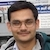# NAEST 2017 Screening Test: Archimedes' PrincipleBy

A body float in a liquid if its weight is less than the buoyant force (upthrust) on it. According to Archimedes' principle, the buoyant force on a body is equal to the weight of the liquid displaced by the body.

## Question from NAEST 2017

Question 1: Force by water on the ball is within

1. 11-12 gm-wt
2. 4-5 gm-wt
3. 15-16 gm-wt

Question 2: Force by the ball on water is within

1. 11-12 gm-wt
2. 4-5 gm-wt
3. 15-16 gm-wt

Question 3: Tension in the thread is
1. 11-12 gm-wt
2. 4-5 gm-wt
3. 15-16 gm-wt

Answer: The weight of the ball is Wb = 15.65 gm-wt and weight of the container with water is Wc = 451.25 gm−wt. Weight of the container when ball is immersed in it is W = 462.72 gm−wt.

The forces acting on the ball are

1. its weight Wb (downwards),
2. the tension in the string T (upwards) and
3. buoyancy force B (upwards).
The net force on the ball is zero (Newton's second law), which gives, \begin{align} W_b = T + B \end{align} The forces on the container and water when ball is immersed in it are
1. weight of the container with water inside it Wc (downwards),
2. force due to ball on water B (downwards) and
3. reaction force on the container from balance W (upwards).
Note that force due to ball on water is equal to force on the ball due to water (Newton's third law). The net force on the container and water is zero (Newton's second law) i.e., \begin{align} W = W_c + B \end{align} which gives \begin{align} B& = W − W_c \\ &= 462.72 − 451.25 \\ &= 11.47\; \text{gm−wt}. \end{align} Substitute in earlier equation to get \begin{align} T &= W_b − B \\ &= 15.65 − 11.47 \\ &= 4.18 \; \text{gm−wt}. \end{align} Thus, option (A) is correct for question 1 and 2, and the option (B) is correct for the question 3.

## Question from NAEST 2014

Question 1: Find the mass of the beaker + water?

Question 2: Find the mass of the hanging object in gram?

Question 3: What is the reading on the dial of the pan balance at this time?

Answer: The reading pan balance with beaker and water is 600 g. Thus, the mass of the beaker and water together is $m_\text{bw} = 600$ g.

The reading of the spring balance with hanging cylindrical mass is 1.4 N. Thus, mg = 1.4, gives \begin{align} m &= 1.4/g = 1.4/10 \\ &= 0.14\, \text{kg} = 140\,\text{g}. \end{align}

When a cylindrical mass is placed in the beaker, reading of the spring balance is 0.2 N. Thus, an upward force, F = 0.2 N, acts on the hanging mass by the spring balance.

Consider cylindrical mass, beaker and water together as a system. The forces acting on this system are

1. weight ($m_\text{bw} + m)g$,
2. force $F$ due to spring balance, and
3. normal reaction $N$ by the pan balance.
Since the system is stationary, Newton's second law gives the resultant force on the system as zero i.e., \begin{align} N +F −(m_\text{bw} +m)g = 0 \end{align} which gives \begin{align} N & = (m_\text{bw} + m)g − F \\ &= (0.600 + 0.140)10 − 0.2 \\ &= 7.2\,\text{N}. \end{align} By Newton's third law, reaction force acting on the pan is also 7.2 N and mass corresponding to this force is \begin{align} m = 7.2/10 = 0.72\, \text{kg} = 720\,\text{g}. \end{align} The readers are encouraged to draw the free body diagram of cylindrical mass and find the buoyant force acting on it.